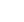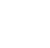# A-level Mathematics for Year 13 - Course 2: General Motion, Moments and Equilibrium, The Normal Distribution, Vectors, Differentiation Methods, Integration Methods and Differential Equations

## Description

This course by Imperial College London is designed to help you develop the skills you need to succeed in your A-level maths exams. You will investigate key topic areas to gain a deeper understanding of the skills and techniques that you can apply throughout your A-level study. These skills include: Fluency – selecting and applying correct methods to answer with speed and efficiency Confidence – critically assessing mathematical methods and investigating ways to apply them Problem solving – analysing the ‘unfamiliar’ and identifying which skills and techniques you require to answer questions Constructing mathematical argument – using mathematical tools such as diagrams, graphs, logical deduction, mathematical symbols, mathematical language, construct mathematical argument and present precisely to others Deep reasoning – analysing and critiquing mathematical techniques, arguments, formulae and proofs to comprehend how they can be applied Over seven modules, covering general motion in a straight line and two dimensions, projectile motion, a model for friction, moments, equilibrium of rigid bodies, vectors, differentiation methods, integration methods and differential equations, your initial skillset will be extended to give a clear understanding of how background knowledge underpins the A -level course. You’ll also be encouraged to consider how what you know fits into the wider mathematical world.

## Specific details

Category of Education Education

## University

Imperial College London

There are no comments posted here yetinfo@educom.net
 About Terms and ConditionsPrivacy PolicyRankingsAccreditations